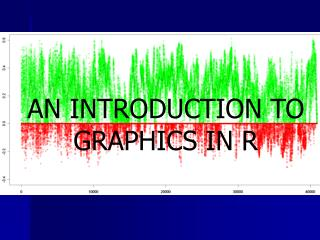DownloadDownload PresentationAN INTRODUCTION TO GRAPHICS IN R

# AN INTRODUCTION TO GRAPHICS IN R

Télécharger la présentation## AN INTRODUCTION TO GRAPHICS IN R

- - - - - - - - - - - - - - - - - - - - - - - - - - - E N D - - - - - - - - - - - - - - - - - - - - - - - - - - -
##### Presentation Transcript

1. AN INTRODUCTION TO GRAPHICS IN R

2. Today • Overview • Gallery of R Graph examples • High-Level Plotting Functions • Low-Level Plotting Functions • Useful functions in conjunction with graphics • Expand your functional toolbox • par • Devices (WE WILL RETURN TO THESE CONCEPTS IN PRACTICAL EXAMPLES) • PRACTICAL/EXPERIMENTATION

3. Overview • One of the best things about R is the ability to create publication-quality graphs • Graph function syntax follows general functional rules of the R language: the fuzzy boundaries between dataset management, statistical analysis, and graphics ultimately is an advantage to the language, but can be a little overwhelming at first. • Corollary: Understanding graphics will facilitate understanding R programming in general---and it gives pretty instant feedback • There are numerous functions available for producing diverse output, with many commonalities in syntax between them • There also are many ways to accomplish a given task. • How a plotting function deals with data often depends on the data type (e.g. matrix, factor, vector) given to it

4. Ooooh….Aaaah…

5. A graph comprised of multiple plots par(“mfrow”=c(2,1)) #change default behavior of plot function to plot 2 graphs per device

6. And more: “multi-bivariate” visualization…

7. Plotting Commands • High-level: create new graphs that inherit properties of one type of plot or another and (e.g. scatterplot) and define graphspace (e.g. x and y boundaries). • Low-Level: additions to existing plots (e.g. lines, additional series, text, shapes) • JUST LIKE OTHER COMMANDS IN R, PLOTTING USES FUNCTIONS THAT TAKE 1 OR MORE ARGUMENTS, WITH PRESET ARGUMENTS THAT CAN BE CHANGED AS DESIRED • par “commands”: default plotting parameters that can be modified to change global behavior of subsequent commands. In essence, you are changing your global preferences for graphing. Some of the par variables can be temporarily changed by adding arguments to high or low level plotting commands; in this case, the par variable is changed for that command only.

8. plot() hist() boxplot() barplot() dotchart() pie() qqplot() qqnorm() pairs() “3D” functions heatmap() image() persp() contour() filled.contour() heatmap.2() Examples of high level plotting commands:

9. points() lines() abline() arrows() segments() rug() … text() mtext() legend() polygon() rectangle() … Low-level plotting commands

10. GRAPH COMMANDS ARE FUNCTIONSand can call other functions • sort() • sum() • density() • lowess() • spline() • ifelse() • …

11. Par • A list of graphical parameters that define the default behavior of all plot functions. • Just like other R objects, par elements are similarly modifiable, with slightly different syntax • e.g. par(“bg”=“lightcyan”) • This would change the background color of all subsequent plots to light cyan • When par elements are modified directly (as above, this changes all subsequent plotting behavior • Some par elements can be modified from within high and low level plotting functions. In this case,

12. Par parameter examples often modifiable from within plotting functions • bg – plot background color • lty – line type (e.g. dot, dash, solid) • lwd – line width • col – color • cex – text size inside plot • mex – text size in margins • mfcol/mfrow – multiple plot option • 2 element vector (#rows,#cols) … many, many more (you tend to learn them as you need them)

13. Add On Packages • A huge number of additional high and low-level plotting functions are available within add-on packages. Genetics and social sciences are particularly well-represented. plot using the enhanced layout capabilities of the lattice package

14. Statistical Function Output • Many statistical functions (regression, cluster analysis) create special objects. Some are special. These arguments will automatically format graphical output in a specific way. • e.g. • lm() • hclust() • agnes()

15. Devices • Specify Destination of Graphics Output • Could be windows in R • Could be files • Not Scalable • JPG • BMP • PNG • Scalable: • Postscript • Pdf • pictex • Others • Win.metafile

16. Practical • Demonstrate Tools (me) • Put them to use to solve silly problems (you)

17. WHO LEFT OUT THE MILK CLUB MILK?

18. FALSE COLOR IMAGERY CHARACTERISTIC SPLATTER PATTERN “MUG SHOT”

19. CRIME SCENE (AERIAL VIEW) OBLIQUE CARTON PLACEMENT: EVIDENCE OF ANTISOCIAL BEHAVIOR PERP’s PEN? MILK SPILL

20. Practical • Make sure that the .Rdata file and the .R file are in the same folder. • In Windows, double-clicking on an .RData file will 1) fire up R, 2) load the objects in the file, and 3) change working directory to the file location. Then open today’s R script. • Alternatively, one can open the R script first, setwd(), and then use the load("RgraphExamples.RData") command in the script to load the objects for analysis